## Digital Modulation

In most media for communication, only a fixed range of frequencies is available for transmission. One way to communicate a message signal whose frequency spectrum does not fall within that fixed frequency range, or one that is otherwise unsuitable for the channel, is to alter a transmittable signal according to the information in your message signal. This alteration is called modulation, and it is the modulated signal that you transmit. The receiver then recovers the original signal through a process called demodulation.

### Digital Modulation Features

#### Modulation Techniques

The Communications Toolbox™ supports these modulation techniques for digital data. All the methods at the far right are implemented in library blocks.Like analog modulation, digital modulation alters a transmittable signal according to the information in a message signal. However, for digital modulation the message signal is restricted to a finite set. Modulation functions output the complex envelope of the modulated signal. Communications Toolbox features enable you to modulate and demodulate signals using various digital modulation techniques. Constellation plots enable you to visualize the constellation diagram of the modulation symbols.

Note

Unless otherwise indicated, the modulation and demodulation functions do not perform pulse shaping or filtering. See Combining Pulse Shaping and Filtering with Modulation for more information about filtering.

The available methods of modulation depend on whether the input signal is analog or digital. The Modulation category lists the digital and analog modulation techniques supported.

Accessing Digital Modulation Blocks.  Open the Modulation library by double-clicking the icon in the main block library. Then open the Digital Baseband sublibrary by double-clicking its icon in the Modulation library.

The Digital Baseband library has sublibraries of its own. Open each of these sublibraries by double-clicking the icon listed in the table below.

Kind of ModulationIcon in Digital Baseband Library
Amplitude modulation AM
Phase modulation PM
Frequency modulation FM
Continuous phase modulation CPM
Trellis-coded modulation TCM

Some digital modulation sublibraries contain blocks that implement specific modulation techniques. These specific-case modulation blocks use the same computational code that their general counterparts use, but provide an interface that is either simpler or more suitable for the specific case. This table lists general modulators along with the conditions under which is general modulator is equivalent to a specific modulator. The situation is analogous for demodulators.

General and Specific Blocks

General ModulatorGeneral Modulator ConditionsSpecific Modulator
General QAM Modulator Baseband

Predefined constellation containing 2K points on a rectangular lattice.

K is the modulation order.

Rectangular QAM Modulator Baseband
M-PSK Modulator Baseband M-ary number parameter is `2`. BPSK Modulator Baseband
M-ary number parameter is `4`. QPSK Modulator Baseband
M-DPSK Modulator Baseband M-ary number parameter is `2`. DBPSK Modulator Baseband
M-ary number parameter is `4`. DQPSK Modulator Baseband
CPM Modulator Baseband M-ary number parameter is `2`, Frequency pulse shape parameter is `Gaussian`. GMSK Modulator Baseband
M-ary number parameter is 2, Frequency pulse shape parameter is `Rectangular`, Pulse length parameter is `1`. MSK Modulator Baseband
Frequency pulse shape parameter is `Rectangular`, Pulse length parameter is `1`. CPFSK Modulator Baseband
General TCM Encoder Predefined signal constellation containing 2K points on a rectangular lattice. Rectangular QAM TCM Encoder
Predefined signal constellation containing 2K points on a circle.M-PSK TCM Encoder

Furthermore, the CPFSK Modulator Baseband block is similar to the M-FSK Modulator Baseband block, when the M-FSK block uses continuous phase transitions. However, the M-FSK features of this product differ from the CPFSK features in their mask interfaces and in the demodulator implementations.

#### Baseband and Passband Simulation

For a given modulation technique, two ways to simulate modulation techniques are called baseband and passband. Baseband simulation, also known as the lowpass equivalent method, requires less computation. The Communications Toolbox supports baseband simulation for digital modulation and passband simulation for analog modulation.

Baseband Modulated Signals Defined

If you use baseband modulation to produce the complex envelope `y` of the modulation of a message signal `x`, then `y` is a complex-valued signal that is related to the output of a passband modulator. If the modulated signal has the waveform

`${Y}_{1}\left(t\right)\sqrt{2}\mathrm{cos}\left(2\pi {f}_{c}t+\theta \right)-{Y}_{2}\left(t\right)\sqrt{2}\mathrm{sin}\left(2\pi {f}_{c}t+\theta \right)\text{\hspace{0.17em}},$`

where fc is the carrier frequency and θ is the carrier signal's initial phase, then a baseband simulation recognizes that this equals the real part of

`$\left[\left({Y}_{1}\left(t\right)+j{Y}_{2}\left(t\right)\right){e}^{j\theta }\right]\mathrm{exp}\left(j2\pi {f}_{c}t\right)\text{\hspace{0.17em}}.$`

and models only the part inside the square brackets. Here j is the square root of -1. The complex vector `y` is a sampling of the complex signal

`$\left({Y}_{1}\left(t\right)+j{Y}_{2}\left(t\right)\right){e}^{j\theta }\text{\hspace{0.17em}}.$`

If you prefer to work with passband signals instead of baseband signals, then you can build functions that convert between the two. Be aware that passband modulation tends to be more computationally intensive than baseband modulation because the carrier signal typically needs to be sampled at a high rate.

#### Modulation Terminology

Modulation is a process by which a carrier signal is altered according to information in a message signal. The carrier frequency, Fc, is the frequency of the carrier signal. The sampling rate is the rate at which the message signal is sampled during the simulation.

The frequency of the carrier signal is usually much greater than the highest frequency of the input message signal. The Nyquist sampling theorem requires that the simulation sampling rate, Fs, be greater than two times the sum of the carrier frequency and the highest frequency of the modulated signal in order for the demodulator to recover the message correctly.

#### Representing Digital Signals

To modulate a signal using digital modulation with an alphabet having M symbols, start with a real message signal whose values are integers from 0 to M-1. Represent the signal by listing its values in a vector, `x`. Alternatively, you can use a matrix to represent a multichannel signal, where each column of the matrix represents one channel.

For example, if the modulation uses an alphabet with eight symbols, then the vector `[2 3 7 1 0 5 5 2 6]'` is a valid single-channel input to the modulator. As a multichannel example, the two-column matrix

```[2 3; 3 3; 7 3; 0 3;] ```
defines a two-channel signal in which the second channel has a constant value of `3`.

### Signals and Delays

All digital modulation blocks process only discrete-time signals and use the baseband representation. The data types of inputs and outputs are depicted in the following figure.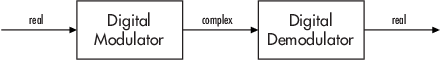Note

If you want to separate the in-phase and quadrature components of the complex modulated signal, use the Complex to Real-Imag (Simulink) block in the Simulink Math Operations library.

#### Integer-Valued Signals and Binary-Valued Signals

Some digital modulation blocks can accept either integer-valued or binary–valued signals. The corresponding demodulation blocks can output either integers or groups of individual bits that represent integers. This section describes how modulation blocks process integer or binary inputs; the case for demodulation blocks is the reverse. You should note that modulation blocks have an Input type parameter and that demodulation blocks have an Output type parameter.

When you set the Input type parameter to `Integer`, the block accepts integer values from `0` to M-`1`. M represents the M-ary number block parameter.

When you set the Input type parameter to `Bit`, the block accepts binary-valued inputs that represent integers. The block collects binary-valued signals into groups of K = log2(M) bits

where

K represents the number of bits per symbol. Since M = 2K, K is commonly referred to as the modulation order.

The input vector length must be an integer multiple of K. In this configuration, the block accepts a group of K bits and maps that group onto a symbol at the block output. The block outputs one modulated symbol for each group of K bits.

#### Constellation Ordering (or Symbol Set Ordering)

Depending on the modulation scheme, the Constellation ordering or Symbol set ordering parameter indicates how the block maps a group of K input bits to a corresponding symbol. When you set the parameter to `Binary`, the block maps [u(1) u(2) ... u(K)] to the integer

`$\sum _{i=1}^{K}u\left(i\right){2}^{K-i}$`

and assumes that this integer is the input value. u(1) is the most significant bit.

If you set M = 8, Constellation ordering (or Symbol set ordering) to `Binary`, and the binary input word is [1 1 0], the block converts [1 1 0] to the integer 6. The block produces the same output when the input is 6 and the Input type parameter is `Integer`.

When you set Constellation ordering (or Symbol set ordering or Symbol mapping) to `Gray`, the block uses a Gray-coded arrangement and assigns binary inputs to points of a predefined Gray-coded signal constellation. The predefined M-ary Gray-coded signal constellation assigns the binary representation

```M = 8; P = [0:M-1]'; de2bi(bitxor(P,floor(P/2)), log2(M),'left-msb') ```

to the `P`th integer.

The following tables show the typical Binary to Gray mapping for M = 8.

Binary to Gray Mapping for Bits

Binary CodeGray Code
000000
001001
010011
011010
100110
101111
110101
111100

Gray to Binary Mapping for Integers

Binary CodeGray Code
00
11
23
32
46
57
65
74

Gray Encoding a Modulated Signal

For the PSK, DPSK, FSK, QAM, and PAM modulation types, Gray constellations are obtained by setting the symbol mapping to Gray-encoding in the corresponding modulation function or System object®.

For modulation functions, you can specify 'gray' for the symbol order input argument to obtain Gray-encoded modulation.

The following example uses the `qammod` function with Gray-encoded symbol mapping.

```y = [0:15]; y = de2bi(y); M = 16; symorder = 'gray'; xmap = qammod(y,M,symorder,'InputType','bit','PlotConstellation',true);```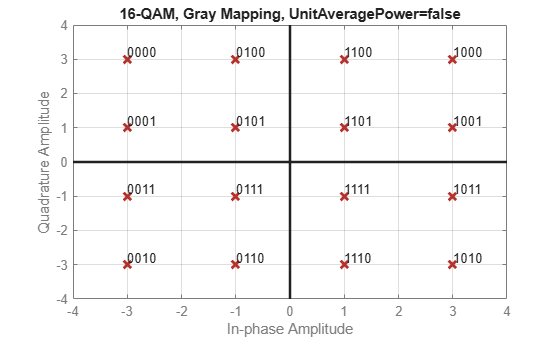Checking the constellation plot, you can see the modulated symbols are Gray-encoded because all adjacent elements differ by only one bit.

#### Upsample Signals and Rate Changes

Some digital modulation blocks can output an upsampled version of the modulated signal, while their corresponding digital demodulation blocks can accept an upsampled version of the modulated signal as input. In both cases, the Rate options parameter represents the upsampling factor, which must be a positive integer. Depending on whether the input signal is single-rate mode or multirate mode, the block either changes the signal's vector size or its sample time, as the following table indicates. Only the OQPSK blocks deviate from the information in the table, in that S is replaced by 2S in the scaling factors.

Process Upsampled Modulated Data (Except OQPSK Method)

Computation TypeInput StatusResult
Modulation Single-rate processingOutput vector length is S times the number of integers or binary words in the input vector. Output sample time equals the input sample time.
Modulation Multirate processingOutput vector is a scalar. Output sample time is 1/S times the input sample time.
Demodulation Single-rate processingNumber of integers or binary words in the output vector is 1/S times the number of samples in the input vector. Output sample time equals the input sample time.
Demodulation Multirate processing

Output signal contains one integer or one binary word. Output sample time is S times the input sample time.

Furthermore, if S > 1 and the demodulator is from the AM, PM, or FM sublibrary, the demodulated signal is delayed by one output sample period. There is no delay if S = 1 or if the demodulator is from the CPM sublibrary.

Illustrations of Size or Rate Changes.  The following schematics illustrate how a modulator (other than OQPSK) upsamples a triplet of frame-based and sample-based integers. In both cases, the Samples per symbol parameter is `2`.The following schematics illustrate how a demodulator (other than OQPSK or one from the CPM sublibrary) processes three doubly sampled symbols using both frame-based and sample-based inputs. In both cases, the Samples per symbol parameter is `2`. The sample-based schematic includes an output delay of one sample period.### PM Modulation

#### DQPSK Signal Constellation Points and Transitions

This model plots the output of the DQPSK Modulator Baseband block. The image shows the possible transitions from each symbol in the DQPSK signal constellation to the next symbol.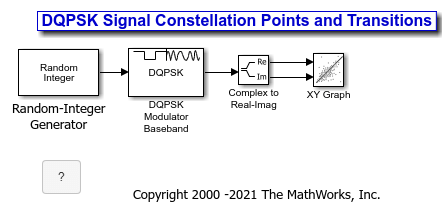To build the model, gather and configure these blocks:

For the Random Integer Generator block, set the M-ary number to 4, set the initial seed to any positive integer scalar (to randomize results you can use the output of the `randn` function), and set the sample time to .01.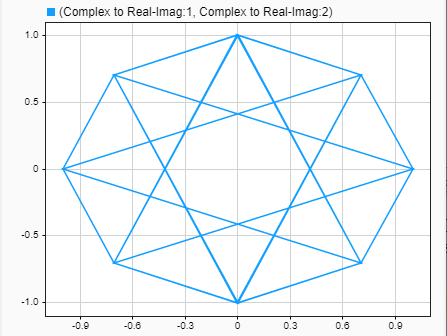The plot illustrates pi/4-DQPSK modulation, because the default Phase offset parameter in the DQPSK Modulator Baseband block is pi/4. To see how the phase offset influences the signal constellation, change the Phase offset parameter in the DQPSK Modulator Baseband block to pi/8 or another value. Run the model again and observe how the plot changes.

### AM Modulation

#### Rectangular QAM Modulation and Scatter Diagram

The model below uses the M-QAM Modulator Baseband block to modulate random data. After passing the symbols through a noisy channel, the model produces a scatter diagram of the noisy data. The diagram suggests what the underlying signal constellation looks like and shows that the noise distorts the modulated signal from the constellation.To open this model, enter `doc_qam_scatter` at the MATLAB command line. To build the model, gather and configure these blocks:

• Random Integer Generator, in the Random Data Sources sublibrary of the Comm Sources library

• Set M-ary number to `16`.

• Set Initial seed to any positive integer scalar, preferably the output of the `randn` function.

• Set Sample time to `.1`.

• Rectangular QAM Modulator Baseband, in the AM sublibrary of the Digital Baseband sublibrary of Modulation

• Set Normalization method to `Peak Power`.

• AWGN Channel, in the Channels library

• Set Es/No to `20`.

• Set Symbol period to `.1`.

• Constellation Diagram, in the Comm Sinks library

• Set Symbols to display to `160`.

Connect the blocks as shown in the preceding figure. On the Simulation tab, in the Simulate section, set Stop time to `250`. The Simulate section appears on multiple tabs.

Running the model produces a scatter diagram like the following one. Your plot might look somewhat different, depending on your Initial seed value in the Random Integer Generator block. Because the modulation technique is 16-QAM, the plot shows 16 clusters of points. If there were no noise, the plot would show the 16 exact constellation points instead of clusters around the constellation points.#### Compute Symbol Error Rate

The example generates a random digital signal, modulates it, adds noise, demodulates the noisy signal, and computes the symbol error rate. The noisy, modulated data is plotted in a constellation diagram. Numerical results and plot may vary due to the random input data.

Create a random digital message and a constellation diagram System object.

```M = 16; % Alphabet size, 16-QAM x = randi([0 M-1],5000,1); cpts = qammod(0:M-1,M); constDiag = comm.ConstellationDiagram('ReferenceConstellation',cpts, ... 'XLimits',[-4 4],'YLimits',[-4 4]);```

Apply 16-QAM modulation and transmit signal through an AWGN channel.

```y = qammod(x,M); ynoisy = awgn(y,15,'measured');```

Demodulate ynoisy to recover the message and check the symbol error rate.

```z = qamdemod(ynoisy,M); [num,rt] = symerr(x,z)```
```num = 79 ```
```rt = 0.0158 ```

Create constellation diagram from noisy data. The signal reference constellation has 16 precisely located points but the transmitted symbols with the noise added causes the scatter plot to have a small cluster of points scattered around each reference constellation point.

`constDiag(ynoisy)`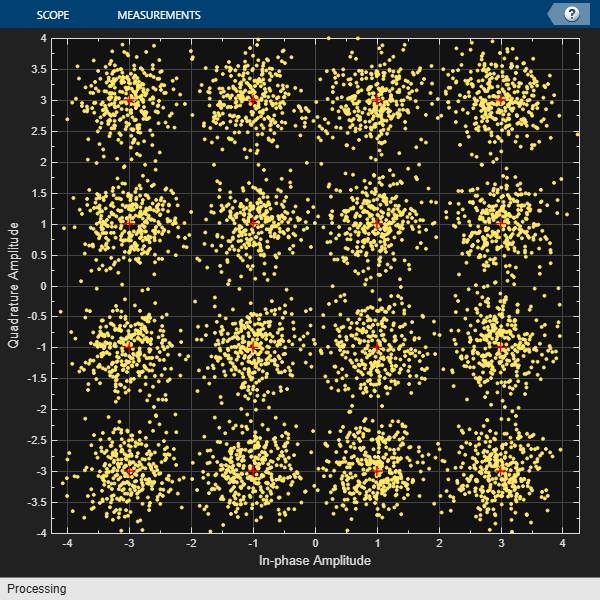#### Combine Pulse Shaping and Filtering with Modulation

Modulation is often followed by pulse shaping, and demodulation is often preceded by a filtering or an integrate-and-dump operation. This section presents an example involving rectangular pulse shaping. For an example that uses raised cosine pulse shaping, see Pulse Shaping Using a Raised Cosine Filter.

Rectangular Pulse Shaping

Rectangular pulse shaping repeats each output from the modulator a fixed number of times to create an upsampled signal. Although it is less realistic than other kinds of pulse shaping, rectangular pulse shaping can be a first step or an exploratory step in algorithm development. If the transmitter upsamples the modulated signal, then the receiver should downsample the received signal before demodulating. The code below uses the `rectpulse` function for rectangular pulse shaping at the transmitter and the `intdump` function for downsampling at the receiver. The “integrate and dump” operation is one way to downsample the received signal.

```% Create a random digital message and a constellation diagram System % object. M = 16; % Alphabet size, 16-QAM x = randi([0 M-1],5000,1); % Message signal Nsamp = 4; % Oversampling rate % Apply 16-QAM modulation and rectangular pulse shaping. Transmit signal % through an AWGN channel. y = qammod(x,M); ypulse = rectpulse(y,Nsamp); ynoisy = awgn(ypulse,15,'measured'); % Downsample at the receiver. ydownsamp = intdump(ynoisy,Nsamp); % Demodulate to recover the message. z = qamdemod(ydownsamp,M);```

### CPM Modulation

#### Phase Tree for Continuous Phase Modulation

This example plots a phase tree associated with a continuous phase modulation scheme. A phase tree is a diagram that superimposes many curves, each of which plots the phase of a modulated signal over time. The distinct curves result from different inputs to the modulator.

This example uses the CPM Modulator Baseband block for its numerical computations. The block is configured using a raised cosine filter pulse shape. The example also illustrates how you can use Simulink and MATLAB together. The example uses MATLAB commands to run a series of simulations with different input signals, to collect the simulation results, and to plot the full data set.

Note

In contrast to this example's approach using both MATLAB and Simulink, the `commcpmphasetree` example produces a phase tree using a Simulink model without additional lines of MATLAB code.To open the model, enter `doc_phasetree` at the MATLAB command line. To build the model, gather and configure these blocks:

• Set Constant value to `s` (which will appear in the MATLAB workspace).

• Set Sampling mode to `Frame-based`.

• Set Frame period to `1`.

• CPM Modulator Baseband

• Set M-ary number to `2`.

• Set Modulation index to `2/3`.

• Set Frequency pulse shape to `Raised Cosine`.

• Set Pulse length to `2`.

• Set Variable name to `x`.

• Set Save format to `Array`.

Do not run the model, because the variable `s` is not yet defined in the MATLAB workspace. Instead, save the model to a folder on your MATLAB path, using the filename `doc_phasetree`.

The second step of this example is to execute the following MATLAB code:

```% Parameters from the CPM Modulator Baseband block M_ary_number = 2; modulation_index = 2/3; pulse_length = 2; samples_per_symbol = 8; L = 5; % Symbols to display pmat = []; for ip_sig = 0:(M_ary_number^L)-1 s = de2bi(ip_sig,L,M_ary_number,'left-msb'); % Apply the mapping of the input symbol to the CPM % symbol 0 -> -(M-1), 1 -> -(M-2), etc. s = 2*s'+1-M_ary_number; sim('doc_phasetree', .9); % Run model to generate x. % Next column of pmat pmat(:,ip_sig+1) = unwrap(angle(x(:))); end; pmat = pmat/(pi*modulation_index); t = (0:L*samples_per_symbol-1)'/samples_per_symbol; plot(t,pmat); figure(gcf); % Plot phase tree.```

This code defines the parameters for the CPM Modulator, applies symbol mapping, and plots the results. Each curve represents a different instance of simulating the CPM Modulator Baseband block with a distinct (constant) input signal.### Exact LLR Algorithm

The log-likelihood ratio (LLR) is the logarithm of the ratio of probabilities of a 0 bit being transmitted versus a 1 bit being transmitted for a received signal. The LLR for a bit b is defined as:

`$L\left(b\right)=\mathrm{log}\left(\frac{\mathrm{Pr}\left(b=0|r=\left(x,y\right)\right)}{\mathrm{Pr}\left(b=1|r=\left(x,y\right)\right)}\right)$`

Assuming equal probability for all symbols, the LLR for an AWGN channel can be expressed as:

`$L\left(b\right)=\mathrm{log}\left(\frac{\sum _{s\in {S}_{0}}{e}^{-\frac{1}{{\sigma }^{2}}\left({\left(x-{s}_{x}\right)}^{2}+{\left(y-{s}_{y}\right)}^{2}\right)}}{\sum _{s\in {S}_{1}}{e}^{-\frac{1}{{\sigma }^{2}}\left({\left(x-{s}_{x}\right)}^{2}+{\left(y-{s}_{y}\right)}^{2}\right)}}\right)$`

where the variables represent the values shown in the following table.

VariableWhat the Variable Represents

`$r$`

Received signal with coordinates (x, y).

`$b$`

Transmitted bit (one of the K bits in an M-ary symbol, assuming all M symbols are equally probable.

`${S}_{0}$`

Ideal symbols or constellation points with bit 0, at the given bit position.

`${S}_{1}$`

Ideal symbols or constellation points with bit 1, at the given bit position.

`${s}_{x}$`

In-phase coordinate of ideal symbol or constellation point.

`${s}_{y}$`

Quadrature coordinate of ideal symbol or constellation point.

`${\sigma }^{2}$`

Noise variance of baseband signal.

`${\sigma }_{x}^{2}$`

Noise variance along in-phase axis.

`${\sigma }_{y}^{2}$`

Note

Noise components along the in-phase and quadrature axes are assumed to be independent and of equal power (i.e., ${\sigma }_{x}^{2}={\sigma }_{y}^{2}={\sigma }^{2}/2$).

### Approximate LLR Algorithm

Approximate LLR is computed by taking into consideration only the nearest constellation point to the received signal with a 0 (or 1) at that bit position, rather than all the constellation points as done in exact LLR. It is defined as :

### Delays in Digital Modulation

Digital modulation and demodulation blocks sometimes incur delays between their inputs and outputs, depending on their configuration and on properties of their signals. The following table lists sources of delay and the situations in which they occur.

Delays Resulting from Digital Modulation or Demodulation

Modulation or Demodulation TypeSituation in Which Delay OccursAmount of Delay
FM demodulatorSample-based processingOne output period
All demodulators in CPM sublibrary Multirate processing, and the model uses a variable-step solver or a fixed-step solver with the Tasking Mode parameter set to `SingleTasking`
D = Traceback length parameter
D+1 output periods
Single-rate processing, D = Traceback depth parameterD output periods
OQPSK demodulatorSingle-rate processing

Multirate processing, and the model uses a fixed-step solver with Tasking Mode parameter set to `Auto` or `MultiTasking`.
Multirate processing processing, and the model uses a variable-step solver or the Tasking Mode parameter is set to Single`Tasking`.
All decoders in TCM sublibrary Operation mode set to `Continuous`, Tr = Traceback depth parameter, and code rate k/n Tr*k output bits

As a result of delays, data that enters a modulation or demodulation block at time T appears in the output at time T+delay. In particular, if your simulation computes error statistics or compares transmitted with received data, it must take the delay into account when performing such computations or comparisons.

#### First Output Sample in DPSK Demodulation

In addition to the delays mentioned above, the M-DPSK, DQPSK, and DBPSK demodulators produce output whose first sample is unrelated to the input. This is related to the differential modulation technique, not the particular implementation of it.

#### Example: Delays from Demodulation

Demodulation in the model below causes the demodulated signal to lag, compared to the unmodulated signal. When computing error statistics, the model accounts for the delay by setting the Error Rate Calculation block's Receive delay parameter to `0`. If the Receive delay parameter had a different value, then the error rate showing at the top of the Display block would be close to 1/2.To open this model, enter `doc_oqpsk_modulation_delay` at the MATLAB command line. To build the model, gather and configure these blocks:

• Random Integer Generator, in the Random Data Sources sublibrary of the Comm Sources library

• Set M-ary number to `4`.

• Set Initial seed to any positive integer scalar.

• OQPSK Modulator Baseband, in the PM sublibrary of the Digital Baseband sublibrary of Modulation

• AWGN Channel, in the Channels library

• Set Es/No to `6`.

• OQPSK Demodulator Baseband, in the PM sublibrary of the Digital Baseband sublibrary of Modulation

• Error Rate Calculation, in the Comm Sinks library

• Set Receive delay to `1`.

• Set Computation delay to `0`.

• Set Output data to `Port`.

• Drag the bottom edge of the icon to make the display big enough for three entries.

Connect the blocks as shown in the preceding figure. On the Simulation tab, in the Simulate section, set Stop time to `1000`. The Simulate section appears on multiple tabs. Then run the model and observe the error rate at the top of the Display block's icon. Your error rate will vary depending on your Initial seed value in the Random Integer Generator block.

### Selected Bibliography for Digital Modulation

 Jeruchim, M. C., P. Balaban, and K. S. Shanmugan, Simulation of Communication Systems, New York, Plenum Press, 1992.

 Proakis, J. G., Digital Communications, 3rd ed., New York, McGraw-Hill, 1995.

 Sklar, B., Digital Communications: Fundamentals and Applications, Englewood Cliffs, NJ, Prentice-Hall, 1988.

 Anderson, J. B., T. Aulin, and C.-E. Sundberg, Digital Phase Modulation, New York, Plenum Press, 1986.

 Biglieri, E., D. Divsalar, P.J. McLane, and M.K. Simon, Introduction to Trellis-Coded Modulation with Applications, New York, Macmillan, 1991.

 Pawula, R.F., “On M-ary DPSK Transmission Over Terrestrial and Satellite Channels,” IEEE Transactions on Communications, Vol. COM-32, July 1984, pp. 752–761.

 Smith, J. G., “Odd-Bit Quadrature Amplitude-Shift Keying,” IEEE Transactions on Communications, Vol. COM-23, March 1975, pp. 385–389.

 Viterbi, A. J., “An Intuitive Justification and a Simplified Implementation of the MAP Decoder for Convolutional Codes,” IEEE Journal on Selected Areas in Communications, vol. 16, No. 2, pp 260–264, Feb. 1998